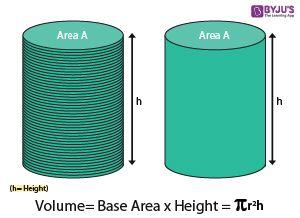Checkout JEE MAINS 2022 Question Paper Analysis : Checkout JEE MAINS 2022 Question Paper Analysis :

# Properties of Cylinder

Before talking about the properties of the cylinder, let us know what a three-dimensional geometry is? Geometry can be divided into two types:

• Plane Geometry
• Solid Geometry

Plane geometry deals with two-dimensional shapes or flat shapes like lines, curves, polygon, etc.that can be drawn on a piece of paper. Solid geometry is about the study of three-dimensional shapes such as cylinders, cubes, spheres, etc. The shapes that are measured in 3 directions are called three-dimensional shapes or solid shapes. The measurements used for three-dimensional shapes are length, width, and height (or depth or thickness). The only difference between 2- dimensional and 3-dimensional shapes is the existence of thickness. These objects are used tremendously in our everyday life. The attributes used in 3D shapes are faces, vertices and edges.

In 3D shapes, the flat surfaces are called faces. The flat surface in which two faces meets is called edge and the point in which three edges meet is called the vertex. Some of the examples of the three-dimensional objects are:

• Cube
• Cuboid
• Prism
• Square Pyramid
• Cylinder
• Cone
• Sphere

Here we will discuss a few properties of a three-dimensional solid object – cylinder in detail.

## Cylinder DefinitionA cylinder is a three-dimensional solid that contains two parallel bases connected by a curved surface. The bases are usually circular in shape. The perpendicular distance between the bases is denoted as the height “h” of the cylinder and “r” is the radius of the cylinder.

## Cylinder Formulas

The lateral Surface area of the cylinder = 2πrh square units

The total surface area of the cylinder, A = 2πr(r+h) square units

The volume of the Cylinder, V = πr2h cubic units.

Where,

“r” is the radius of the cylinder

“h” is the height of the cylinder

## Cylinder Properties

Some of the important properties of the cylinder are as follows:

• The bases of the cylinder are always congruent and parallel to each other.
• If the axis of the cylinder is a right angle to the base and the bases are exactly over each other, then it is called as “Right Cylinder”.
• If one of the bases of the cylinder is displayed sideways, and the axis does not produce the right angle to the bases, then it is called “Oblique Cylinder”.
• If the bases are circular, then it is called a right circular cylinder.
• The best alternative to the circular base of a cylinder is an ellipse. If the base of the cylinder is elliptical in shape, then it is called an “Elliptical Cylinder”.
• If the locus of a line moving parallel and fixed distance from the axis, a circular cylinder is produced.
• A cylinder is similar to the prism since it has the same cross-section everywhere.

## Sample Problem

The sample example for the cylinder is given below.

Question: Find the volume of the cylinder with the radius of 5cm and height 7 cm?

Solution:

Given:

Height,h = 7 cm

Volume of the cylinder, V = πr2h cubic units.

V = (22/7) x 52 x 7

V = 22 x 25

V= 550 Cubic units.

Therefore the volume of the cylinder is 550 cm3.

For more such interesting information on properties of the cylinder and other quadrilaterals, register with BYJU’S – The Learning App and also watch videos to learn with ease.

 Related Links Properties of Quadrilaterals Properties of Circle Properties of Parallelogram Properties of Rhombus
Test your knowledge on Properties Of Cylinder